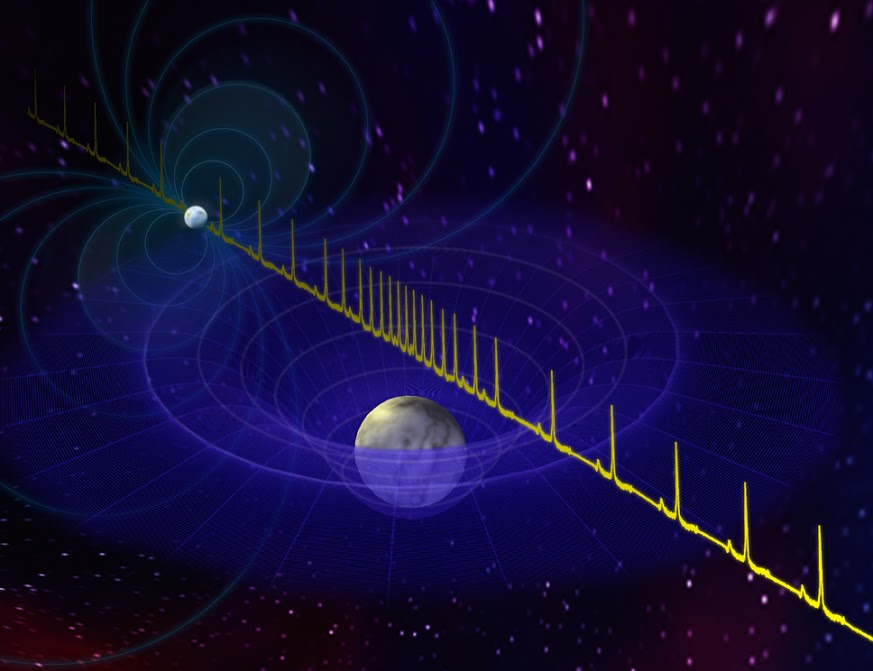# Flight Delay

In Relativity by Brian Koberlein1 Comment

It’s a well known law of physics that the speed of light (in a vacuum) is always the same, regardless of your frame of reference (essentially your vantage point). But this isn’t entirely true. It actually depends on how you define “speed”.

There are different ways to define speed, which is easy to see if you think about a car. One way to determine the speed of a car would be to look at the speedometer. If it says you are travelling 50 miles per hour (80 kilometers per hour), then that is the car’s speed at that moment in time. We sometimes call this the instantaneous speed. Another way to determine the car’s speed is to time how long it takes the car to travel a certain distance. So if we observe the car travel 50 miles in one hour, then we would say its speed if 50 mph. This is known as an average speed.

So what does this have to do with the speed of light? After all, if the speed of light is constant, doesn’t that mean the instantaneous speed and average speed will always be the same? They would be if light always travelled in a straight line (in flat space), but we know that space is actually curved. Gravity is the result of the curvature of space and time around a mass, so near a large mass like the Sun space is not flat. This means the average speed isn’t necessarily the same as the instantaneous speed.

We can see this with the car example again. Suppose two towns were 100 miles apart, and connected by a perfectly straight road. Then suppose we travel in a car with cruise control set at exactly 50 mph. The instantaneous velocity of the car is constant, so we would expect the travel time between the cities to be exactly 2 hours. But halfway on our journey, there is a bit of construction, so we must take a slight detour. Our speed remains 50 mph, but because of the detour our path is not perfectly straight. So instead of 2 hours, it takes us a bit longer, say 2 hours and 4 minutes. Thus the average speed is 48.4 mph.

We see this same effect with light. The mass of the Sun warps space near it, therefore light passing near the Sun has a slight detour. That means light from a planet on the other side of the solar system from earth reaches us a tiny bit later than we would otherwise expect. The first measurement of this time delay was in the late 1960s by Irwin Shapiro. Radio signals were bounced off Venus from Earth when the two planets were almost on opposite sides of the sun. The measured delay of the signals’ round trip was about 200 microseconds, just as predicted by general relativity. This effect is now known as the Shapiro time delay, and it means the average speed of light (as determined by the travel time) is slightly slower than the (always constant) instantaneous speed.

This effect has been used to determine the mass of certain neutron stars. In 2010, as described in a paper from Nature, a binary system consisting of a neutron star and a white dwarf was observed. The neutron star is a pulsar, meaning that it sweeps out a beam of intense energy as it rotates (kind of like a lighthouse). We see this as repeating radio pulses.

The two stars orbit each other in such a way that the white dwarf almost passes in front of the pulsar each orbit. This means the radio pulses pass close to the white dwarf before reaching us, and experiences a Shapiro time delay. Since the amount of the delay depends on the amount of spatial warping, which depends on the mass of the white dwarf, the team could use the delay to determine the mass of the white dwarf. Knowing that, they could determine the mass of the neutron star.

What they found was that this particular neutron star (PSR J1614-2230) is the most massive neutron star currently known, with a mass of about twice the Sun. It is so massive that we aren’t entirely sure how it exists.

In our everyday lives, a flight delay can be a bit of an annoyance. For light, a flight delay is a useful astronomical tool.

1.